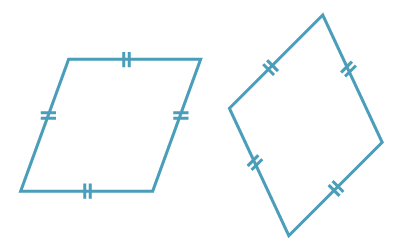## Rhombuses

######## Definition of a rhombus
• A rhombus is a quadrilateral with all sides equal.
##### Properties of a rhombus
• The opposite sides of a rhombus are parallel.
• The opposite angles of a rhombus are equal.
• The diagonals of a rhombus bisect each vertex angle.
• The diagonals of a rhombus bisect each other at right angles.
##### Tests for a rhombus

A quadrilateral is a rhombus if:

• it is a parallelogram, and a pair of adjacent sides are equal, or
• its diagonals bisect each other at right angles, or
• its diagonals bisect each vertex angle.